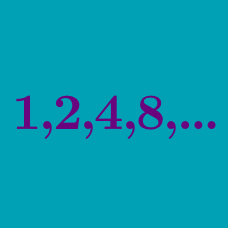Algebra

# Arithmetic and Geometric Progressions Problem Solving

Let $\{a_n\}$ be a geometric progression such that $a_1+a_3=15$ and $a_2+a_4=30$. What is the sum of the first $5$ terms of this geometric progression?

The sum of three numbers in geometric progression is $52$. If the product of the three numbers is $1728$, what is the value of the maximum number?

Let $\{a_n\}$ be a geometric progression such that $a_5=\frac{15}{16} \mbox{ and } a_8=\frac{15}{128}.$ What is the smallest integer $n$ for which $a_n < 0.001$?

The curve $y=-x^3+8x^2+40x$ and the line $y=k$ intersect at $3$ distinct points. If the $x$-coordinates of these $3$ points form a geometric progression, what is the real number $k?$

$3$ real numbers that form a geometric progression have sum equal to $175$ and product equal to $17576$. What is the sum of the largest and smallest numbers?

×